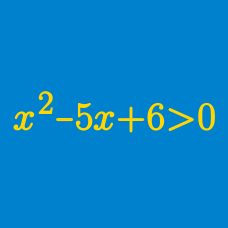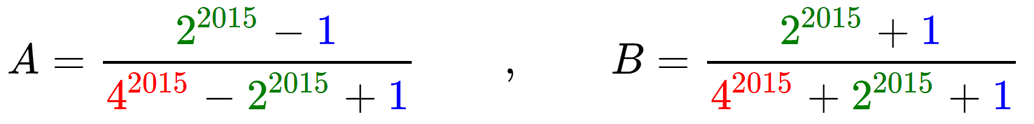Algebra

# Polynomial Inequalities Problem Solving

If $x+y=20$, what is the maximum value of $2xy$?

A rectangular wall for a chicken coop is to be made out of a $44 \mbox{ m}$ wire mesh. Let $x$ be the shorter side of the rectangle in meters. If the area of the rectangle, $A$, is $120 \mbox{ m}^2 < A < 121 \mbox{ m}^2$, then $x$ must satisfy $a < x < b$. What is the value of $a+b$?

If $x$ and $y$ are positive numbers, what is the minimum value of $(3x + 4y)\left(\frac{27}{x} + \frac{4}{y} \right)$?Which is larger?

Hint: Let $x = 2 ^ {2015}$.

What is the maximum value of $x$ that satisfies the inequality $(x - 6)(x^2 - 4x + 9) \leq 0$?

×